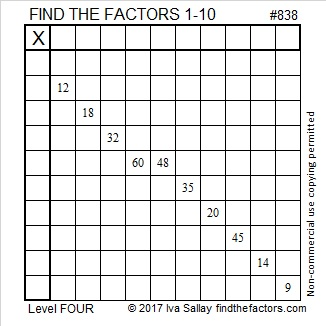# In Which Bases is 838 a Palindrome?

838 is a palindrome in base 10. Is it a palindrome in any other bases? Yes, two others.

• 262 BASE 19 because 2(19²) + 6(19¹) + 2(19º) = 838
• 141 BASE 27 because 1(27²) + 4(27¹) + 1(27º) = 838

There is only one way 838 can be written as the sum of consecutive numbers:

• 208 + 209 + 210 + 211 = 838Print the puzzles or type the solution on this excel file: 10-factors-835-842

• 838 is a composite number.
• Prime factorization: 838 = 2 × 419
• The exponents in the prime factorization are 1 and 1. Adding one to each and multiplying we get (1 + 1)(1 + 1) = 2 × 2 = 4. Therefore 838 has exactly 4 factors.
• Factors of 838: 1, 2, 419, 838
• Factor pairs: 838 = 1 × 838 or 2 × 419
• 838 has no square factors that allow its square root to be simplified. √838 ≈ 28.9482This site uses Akismet to reduce spam. Learn how your comment data is processed.